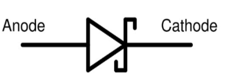Engineering Jobs   »   uprvunl je electrical quiz

# UPRVUNL’21 EE: Daily Practices Quiz 27-July-2021

Each question carries 1 mark.
Negative marking: 1/4 mark
Total Questions: 06
Time: 08 min

Q1. Failure of insulation on stator winding of generator results in
(a) Short circuit between phases
(b) Short circuit between turns
(c) Short circuit between one or more phases and earth
(d) All of the above

Q2. A Schottky diode is
(a) A minority carrier device
(b) A majority carrier device
(c) Both majority and minority carrier device
(d) A fast recovery diode

Q3. Which of the following cannot be measured by Multimeter?
(a) Voltage
(b) Current
(c) Resistance
(d) Frequency

Q4. A centrifugal switch is provided for disconnecting the auxiliary winding in a
(a) Capacitor start motor
(b) Capacitor run motor
(c) Hysteresis motor
(d) Reluctance motor

Q5. The ratio of primary to secondary voltages is 2:1. The saving in terms of weight of copper required if an autotransformer is used instead of a two-winding transformer will be:
(a) 33.3 %
(b) 50 %
(c) 66.7 %
(d) 95 %

Q6. Fusing factor of fuse is always
(a) More than 1
(b) Less than 1
(c) Infinity
(d) Zero

SOLUTIONS

S1. Ans.(d)
Sol. Failure of insulation on stator winding of generator causes:
Inter-turn faults involving turns of the same phase winding
Phase-to-earth fault
Phase-to-phase faults

S2. Ans.(b)
Sol. Schottky diode:It is a semiconductor diode formed by the junction of a semiconductor with a metal.
The Schottky barrier diode current condition is through majority carriers, which are electrons in an N-type semiconductor.
It has a low forward voltage drop and a very fast switching action.

S3. Ans.(d)
Sol. A typical Multimeter can measure current, voltage and resistance.

S4. Ans.(a)
Sol. In case of capacitor start motor, a centrifugal switch is provided for disconnecting the auxiliary winding when the motor attains speed up-to 70-80% of synchronous speed.

S5. Ans.(b)
Sol. Here, a=2
∴ Cu saving=1/a=1/2=0.5=50%

S6. Ans.(a)
Sol. Fusing factor=(minimum fusing current)/(current rating of fuse)
Fusing factor of fuse is always >1.

Sharing is caring!